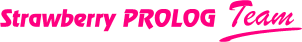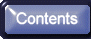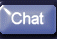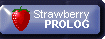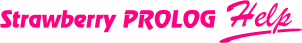What is specific in Strawberry Prolog syntax?

This compiler supports all the features of the standard (or will support them soon) but also gives many possibilities which you have not with another Prolog compiler. Our philosophy was to give you maximal freedom and let you do as you like best. There is another philosophy, which says that if one's hands are tied, mistakes will be fewer. We do not share this thesis, and made an extension of the language which allow many constructions which other Prolog compilers would understand as syntax errors.

Here are the differences:

Operators

In Strawberry Prolog you cannot put operators in apostrophes. When one operator is in apostrophes then it will be accepted as an atom not as an operator. So, you cannot have an operator like 'A' because this atom cannot be written without apostrophes. So if you write a '+' b or +(a,b) then this will give a syntax error. The correct will be a + b and '+'(a,b) respectively. Notice that if you want to use the prefix notation then you should to put the operator in apostrophes because +(a,b) is syntax error (i.e. it is the same as + (a,b) or the space between the operator and the bracket is not important). Anyway the space between the term and the bracket is important and '+'(a,b) is not the same as '+' (a,b).

Lists and functors

In the Standard, you can write a list using square brackets '[' and ']'. For example:
[] - the empty list.
[a, b, c] - list of elements a, b, c .
[X|Y] - list with head X and tail Y .
[a, b | T] - list beginning with elements a, b and tail T .

In Strawberry Prolog you can write every of the above lists. You can write even a list like:
[ | T] - list beginning with nothing, its tail being T , same as T . Of course, this is an absolutely useless extension.

In the Standard you can write a functor with arguments like this:
f( a, b, c) .

In Strawberry Prolog you have a functor and a list of arguments. This means that you can type between ( and ) everything you can type between [ and ] . For example:
f() - f with an empty list of arguments.
f( a, b | T) - f with its argument list beginning with elements a, b and tail T .
f( | T) - f with T as its argument list.

The place of the functor can be occupied by a variable too:
X( a) - functor X (variable) with argument a .
X( | Y) - functor X (variable) with list of arguments Y (variable).

This makes extremely easy to define the operator =.. in Strawberry Prolog. Here is the definition:
X(|Y)=..[X|Y].

Remark: You must have no space between the functor and the ( . This is so both here and in the Standard.

Atoms

In Strawberry Prolog the atoms are represented as chain of constants. For example:
abc is equivalent to like_atom(a, like_atom(b , c)).

The functor like_atom is special built-in functor which do the internal representation of the atoms. You don't need to use like_atom functor unless you want to make some strange pattern (unification condition) like these:
like_atom(a, like_atom(_, c)) - which will be unifiable with every atom of three characters, the first one being a , and last one c .
like_atom(_, bc) - which will be unifiable with every atom of three characters that ends with bc .
like_atom(a, like_atom(b, _)) - which will be unifiable with every atom of three or more characters that starts with ab .

Unfortunately, you can not write a pattern that is unifiable with all the atoms with some given end.

As an example, try running the program:
?- X=c, write( like_atom(a, like_atom(b, X)) ).

Change X=c with X=[c] , X=f(c) and X=cd .

Internal functors

In Strawberry Prolog there are three internal functors:
like_atom(Constant, Atom), Functor( | List_of_Arguments) and [Head | Tail].

Is it possible to write a term where the functor is not an atom? For example [a](b,c). This strange construction will give you a syntax error but you still can make such a term in this way:
like_functor([a], [b,c]) or F=[a], F(b,c). The second variant is simpler but it is not a syntax construction. Anyway this is the way you can construct such term in the time of program execution.

So, in Strawberry Prolog you can use the built-in functors like_atom and like_functor in order to construct some strange terms. Also the system uses these functors in order to write these strange terms which your program accidentally constructed.

We have also one built-in constant which we use for similar purposes. This constant is like_set_const and we use it for constructions of the type: {a, b, c}. For example the empty set {} will be represented as like_functor(like_set_const, []) or as F=like_set_const, F().